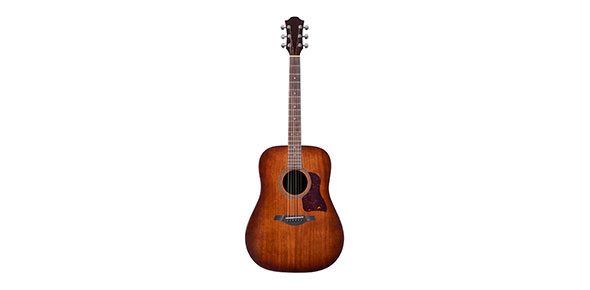# Time Signature; Incorrect Measure

4 QuestionsSettingsChoose the measure that is WRONG. Measure 1 is B Measure 2 is C Measure 3 is D Measure 4 is E If all measures are correct click A for your answer.

• 1.
• A.

B

• B.

C

• C.

D

• D.

E

• E.

A

• 2.
• A.

B

• B.

C

• C.

D

• D.

E

• E.

A

• 3.
• A.

B

• B.

C

• C.

D

• D.

E

• E.

A

• 4.
• A.

B

• B.

C

• C.

D

• D.

E

• E.

A

Related Topics